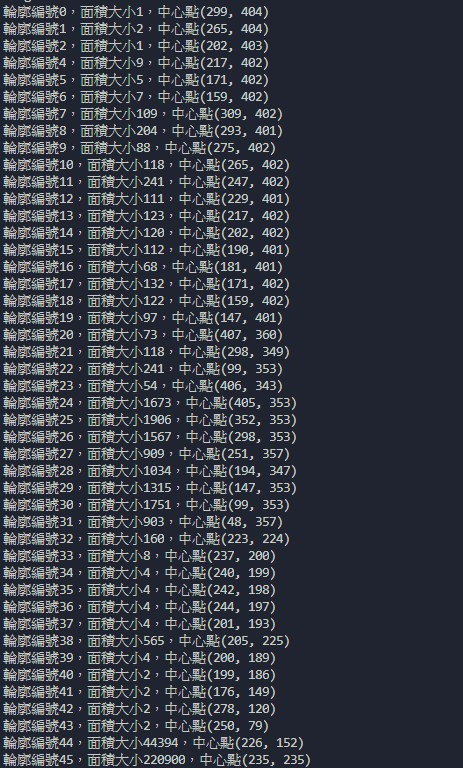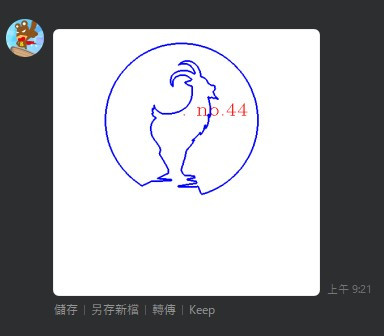#DAY 14
0

## Contours特徵數值(面積、中心點)

``````#將二值化的圖片放到findContours()中
contours,hierarchy = cv.findContours(binary,cv.RETR_LIST,cv.CHAIN_APPROX_SIMPLE)

n = len(contours)
for i in range(n):
M = cv.moments(contours[i])
area = M['m00']
cx = int(M['m10']/M['m00'])#三角形的x範圍  800-2200
cy = int(M['m01']/M['m00'])#三角形的y範圍  0-3800
center = tuple((cx,cy))
print('輪廓編號%d，面積大小%d，中心點%s'%(i,area,str(center)))
``````## 以Contour特徵標定ROI

``````#建立空白圖片
empty = np.ones(img.shape,dtype=np.uint8)*255

total_area = img.shape*img.shape
font = cv.FONT_HERSHEY_COMPLEX
print(total_area)
n = len(contours)
for i in range(n):
M = cv.moments(contours[i])
area = M['m00']
if area/total_area>0.15 and area/total_area<0.50:#若面積大於總面積的15%且小於50%
cx = int(M['m10']/M['m00'])
cy = int(M['m01']/M['m00'])
center = tuple((cx,cy))
if cx>img.shape*0.25 and cx<img.shape*0.75:
cv.putText(empty,'. no.='+str(i),center,font,2,(0,0,255),1)
print('輪廓編號%d，面積大小%d，中心點%s'%(i,area,str(center)))
#將輪廓描繪在複製圖上
copy = cv.drawContours(empty,contours[i],-1,(255,0,0),2)
``````

1.要標定文字的圖片
2.要標定的文字內容
3.標定的座標位置
4.字型設定
5.字體大小
6.字體顏色
7.字體粗細